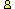CTK Exchange CTK Wiki Math Front Page Movie shortcuts Personal info Awards Terms of use Privacy Policy Cut The Knot! MSET99 Talk Games & Puzzles Arithmetic/Algebra Geometry Probability Eye Opener Analog Gadgets Inventor's Paradox Did you know?... Proofs Math as Language Things Impossible My Logo Math Poll Other Math sit's Guest book News sit's Recommend this siteCTK Exchange

 Subject: "Question on barycentric coordinates" Previous Topic | Next Topic
 ConferencesThe CTK ExchangeThis and thatTopic #889Printer-friendly copyEmail this topic to a friend Reading Topic #889
alphabravo112
Member since Mar-24-09
Mar-24-09, 06:59 AM (EST)"Question on barycentric coordinates"

 Suppose, we have a triangle A(x1,y1), B(x2,y2) and C(x3,y3). There is a point inside the triangle P(xp,yp), thenxp = w1*x1 + w2*x2 + w3*x3yp = w1*y1 + w2*y2 + w3*y3where w1,w2,w3 are barycentric coordinates such that 0<=wi<=1 and w1+w2+w3 = 1.I can compute w1, w2, w3 by w1 = area(BCP)/area(ABC), w2 = area(ACP)/area(ABC) and w3 = area(ABP)/area(ABC). These areas can be computed using point-point distances (a total of 6) and using Heron's formula (or Cayley Menger determinants if I consider a 3-d problem with 4 points and 5th unknown lying inside the convex hull (tetrahedron) of the four).Question: I will give you a brief scope of the problem. Suppose A, B and C are some devices which have GPS device so they know their exact locations (xi's,yi's) and we wish to determine the location of P. If I know the locations of A, B and C and the six inter-node distances, I can solve for xp and yp using a linear equation. I need the distances to compute areas (and, in turn barycenters). Is there any other technique to compute these barycenters, for instance, using only the angles in the four triangles generated by A,B,C,P. or some other weighting technique.I know area formula using radius of circumscribed circle and sine of angles but that won't help coz of the radius. My hope is that since barycenters are ratios they should be indifferent to distances, or radii for that matter. coz the barycenters wont change if I scale the coordinates appropriately (???). so there must be some representation with orientation or angles ?This question arises in a research problem I am addressing in the context of localization (finding positions) of points in m-dimensional Euclidean spaces.

Alert | IP Printer-friendly page | Reply | Reply With Quote | Top
alexbCharter Member
2345 posts
Mar-24-09, 08:35 AM (EST)1. "RE: Question on barycentric coordinates"
In response to message #0

 I am not sure I understand the problem. I'll ask a couple of questions:>Question: I will give you a brief scope of the problem. >Suppose A, B and C are some devices which have GPS device so >they know their exact locations (xi's,yi's) and we wish to >determine the location of P. If I know the locations of A, B >and C and the six inter-node distances, I can solve for xp >and yp using a linear equation. What are the six inter-node distances, or the nodes themselves, for that matter?>I need the distances to compute areas (and, in turn >barycenters). Is there any other technique to compute these >barycenters, for instance, using only the angles in the four >triangles generated by A,B,C,P. or some other weighting >technique. Are you assuming that Earth is flat? Given that you mention the GPS, ignoring the curvature appears, say, light hearted.>>I know area formula using radius of circumscribed circle and >sine of angles but that won't help coz of the radius. My >hope is that since barycenters are ratios But sine is also a ratio.>... they should be >indifferent to distances, or radii for that matter. coz the >barycenters wont change if I scale the coordinates >appropriately (???). This is why I asked about the curvature. Scaling is less meaningful on a sphere than on a plane.>so there must be some representation >with orientation or angles ? There is a trigonometric form of Ceva's theorem that might be relavant to your inquiry:https://www.cut-the-knot.org/triangle/TrigCeva.shtml

Alert | IP Printer-friendly page | Reply | Reply With Quote | Top

 Conferences | Forums | Topics | Previous Topic | Next Topic
 Select another forum or conference Lobby The CTK Exchange (Conference)   |--Early math (Public)   |--Middle school (Public)   |--High school (Public)   |--College math (Public)   |--This and that (Public)   |--Guest book (Protected)   |--Thoughts and Suggestions (Public) Educational Press (Conference)   |--No Child Left Behind (Public)   |--Math Wars (Public)   |--Mathematics and general education (Public)You may be curious to have a look at the old CTK Exchange archive.
Please do not post there.